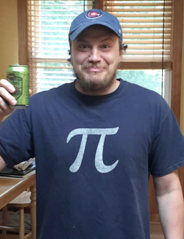# How often does the best team win?

Benjamin Baumer & Michael Lopez (with Gregory J Matthews)

## How often does the best team win?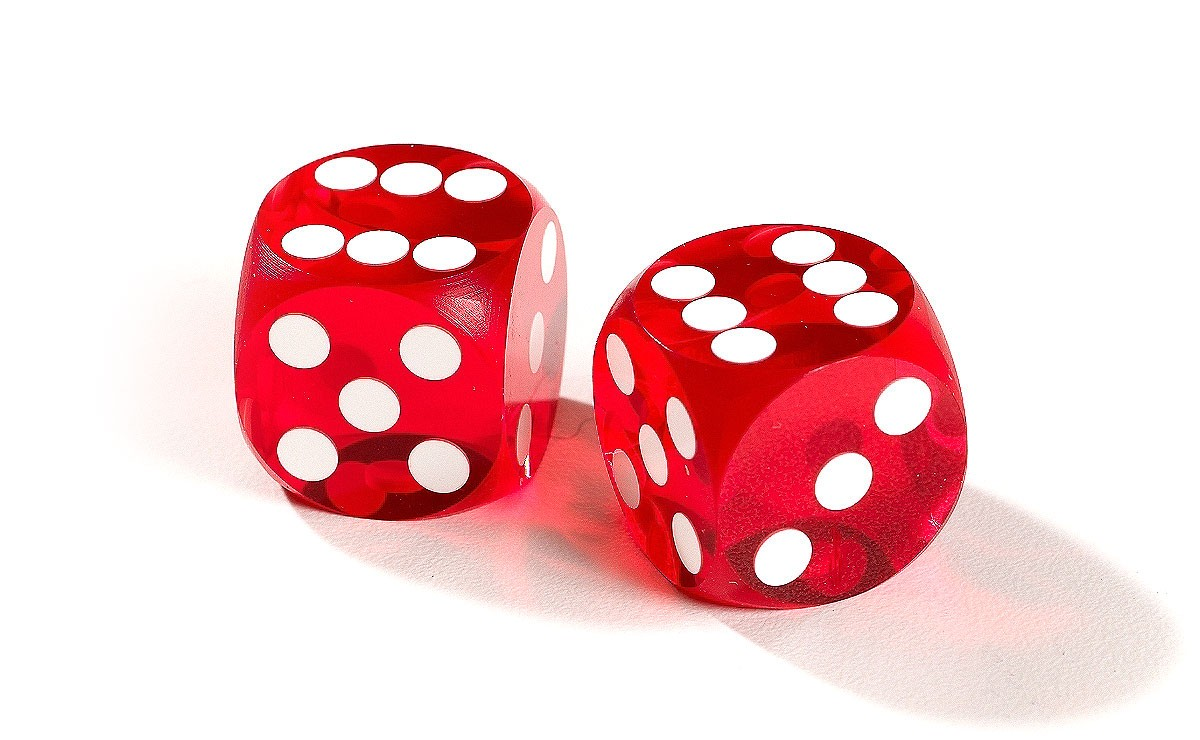Michael Lopez, Gregory J Matthews, Benjamin Baumer
https://github.com/bigfour/competitiveness

## What we remember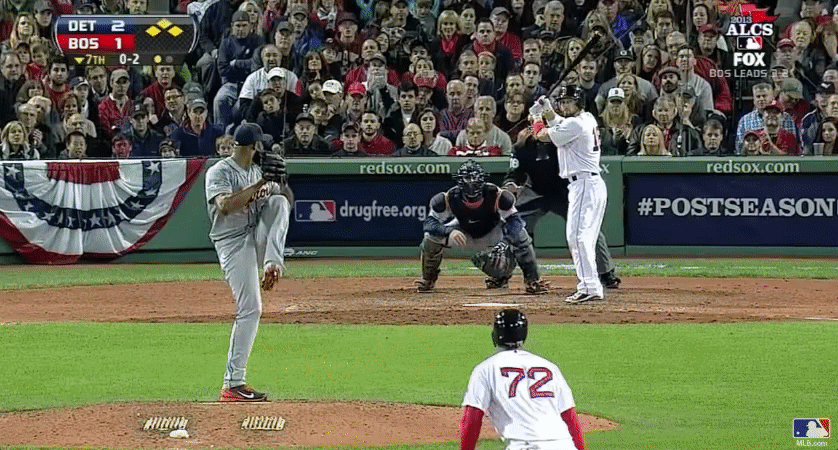## What we forget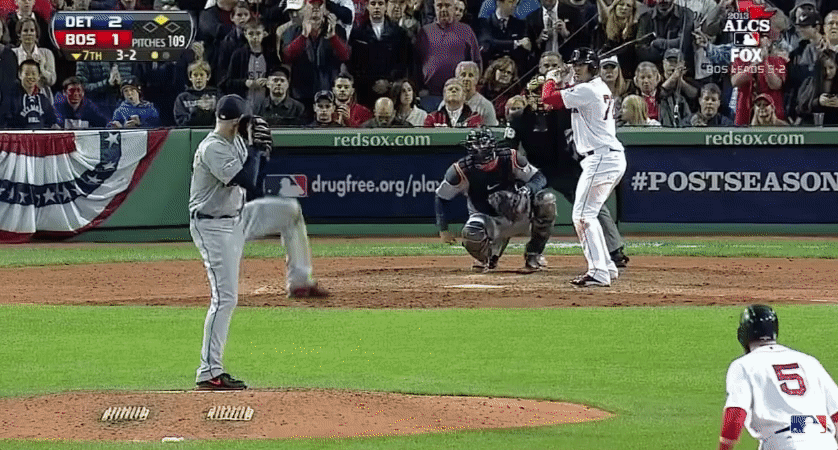## Pitches in the series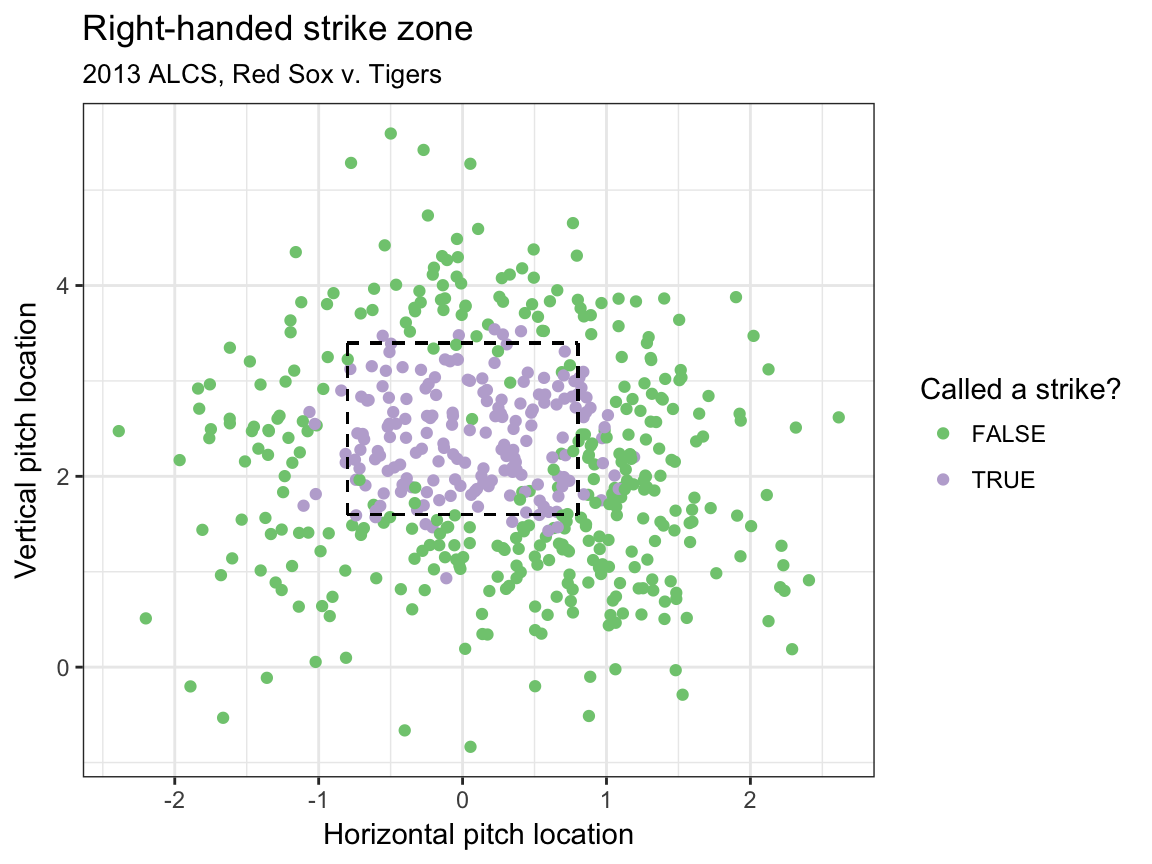## That pitch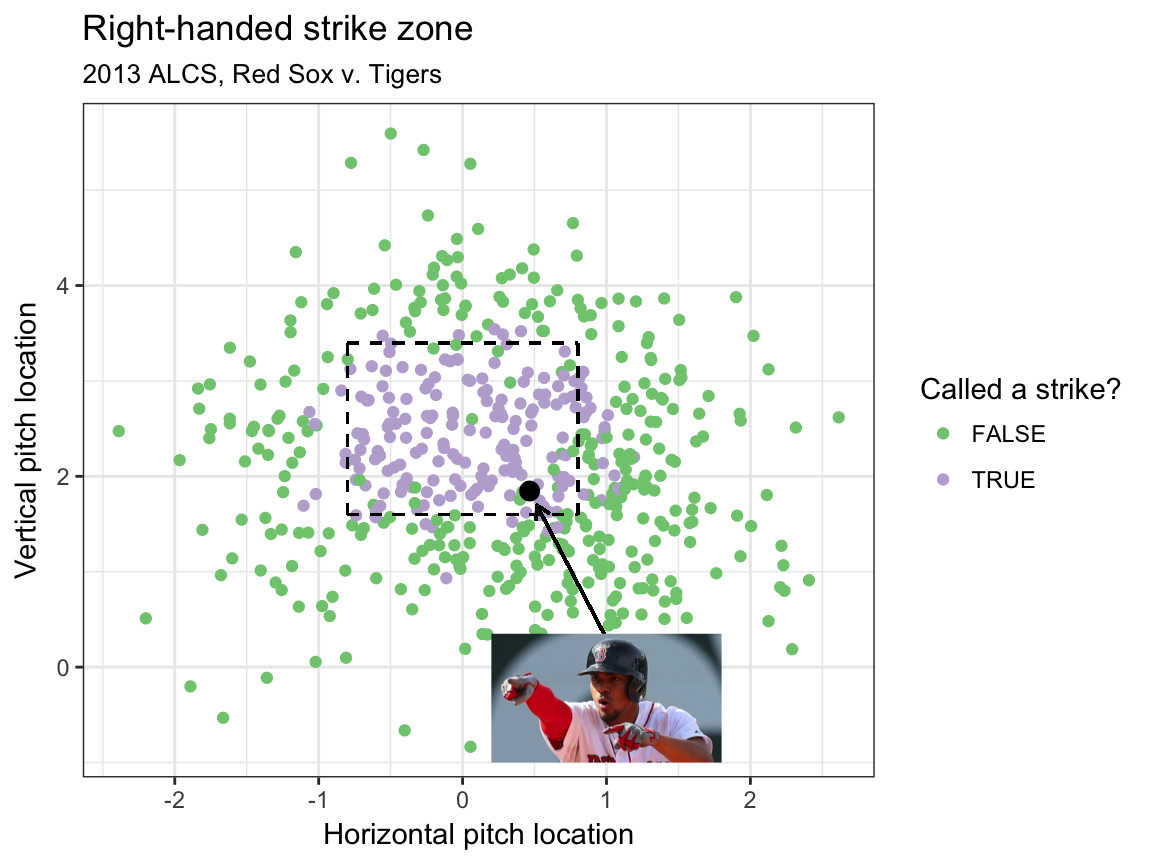## The truth is…

• About 80% of pitches taken at this location called a strike (using advanced modeling)
• Walk worth about 12% in win expectency (32% to 44%)
• Red Sox were lucky!

## What we also forget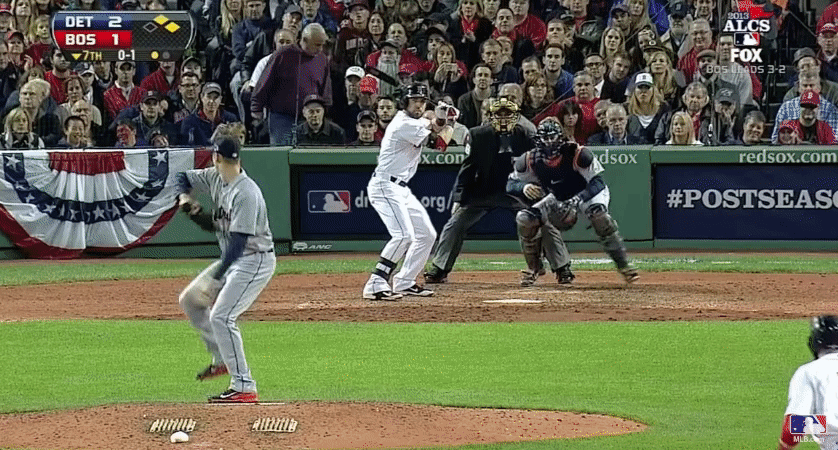• Jose Iglesias: 98.5% fielding percentage (Note: Statcast data unavailable)
• Error worth about 20% in win expectency over out at second (36% to 56%)
• Red Sox were lucky!

## How to define parity

the state or condition of being equal

## What parity looks like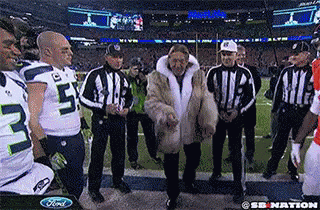## What parity does not look like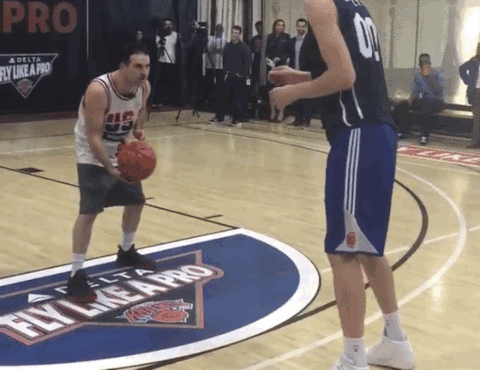## Parity checklist

1. Equality at a fixed time
2. Within season equality
3. Between season equality

## Prior work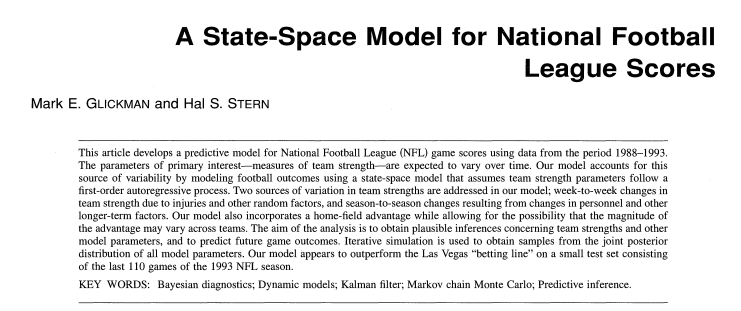## Challenges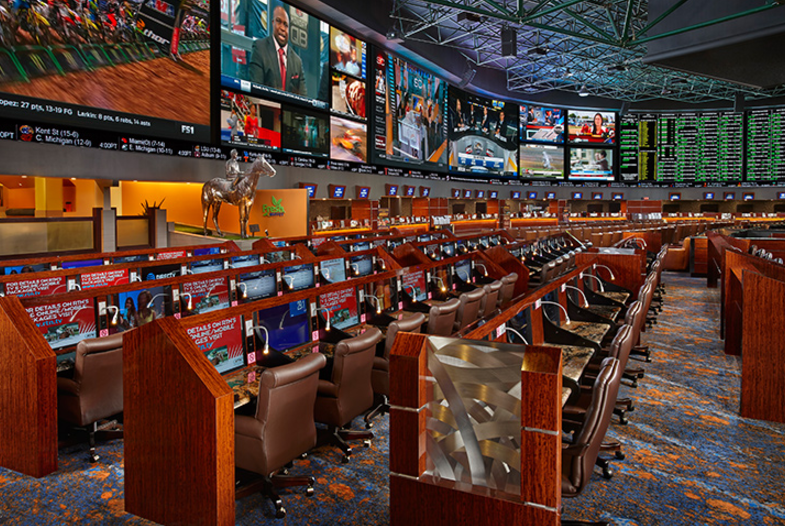## Moneylines

Team Line ($$\ell$$) Probability ($$p$$) Normalized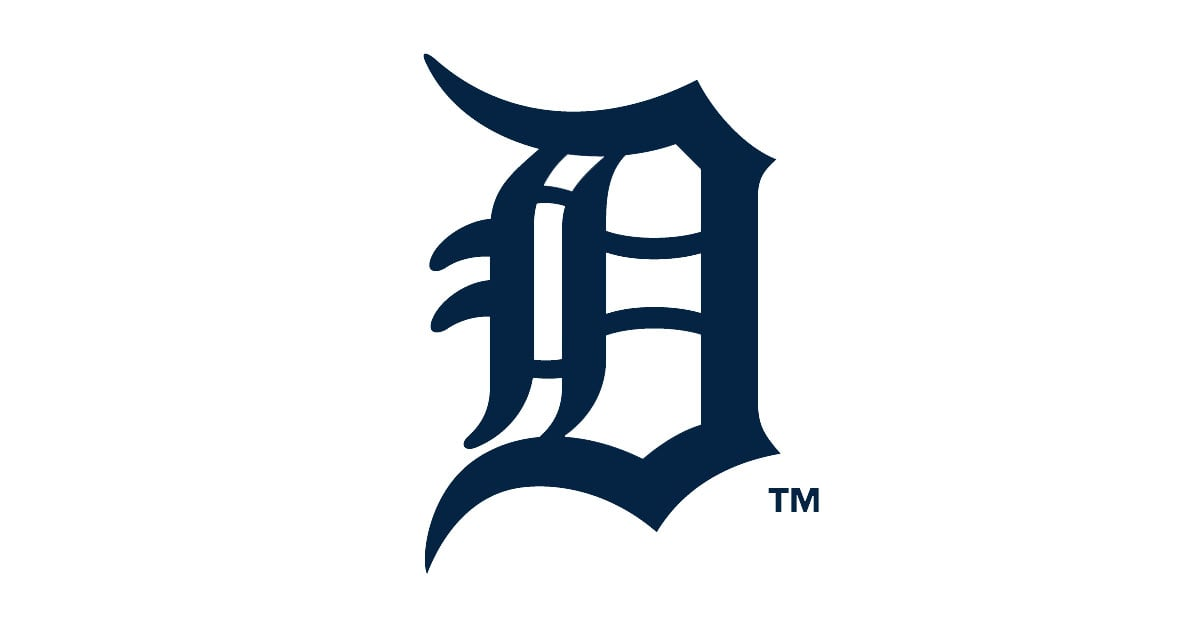-127 0.559 0.548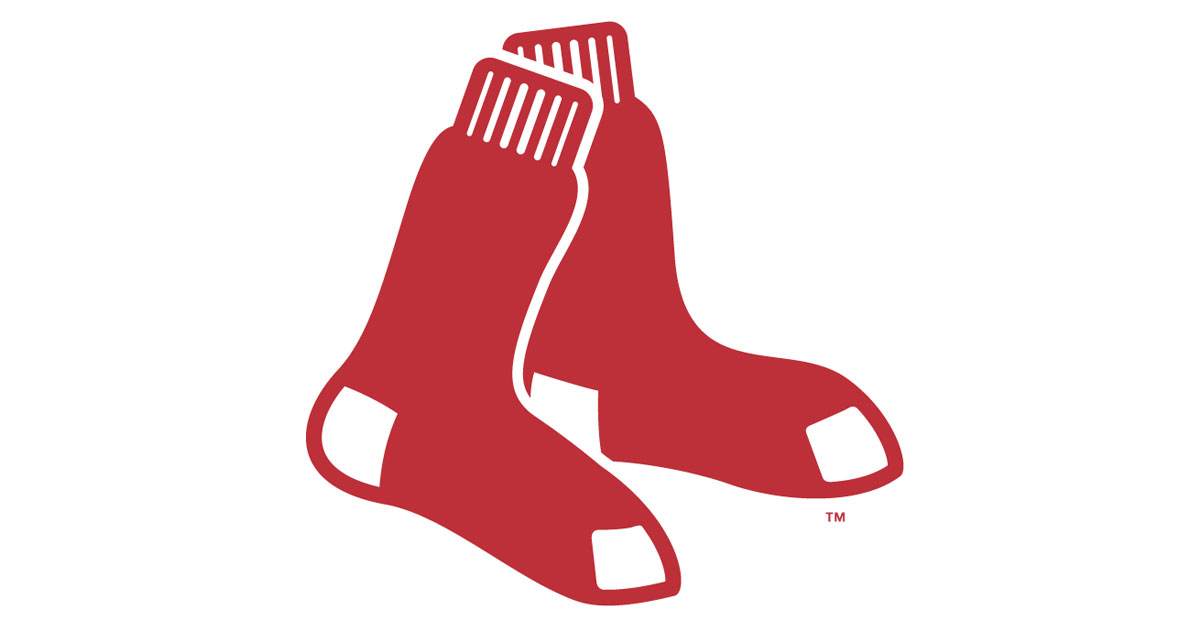+117 0.461 0.452

$p_i(\ell_i) = \begin{cases} \frac{100}{100 + \ell_i} & \text{ if } \ell_i \geq 100 \\ \frac{|\ell_i|}{100 + |\ell_i|} & \text{ if } \ell_i \leq -100 \end{cases} \,.$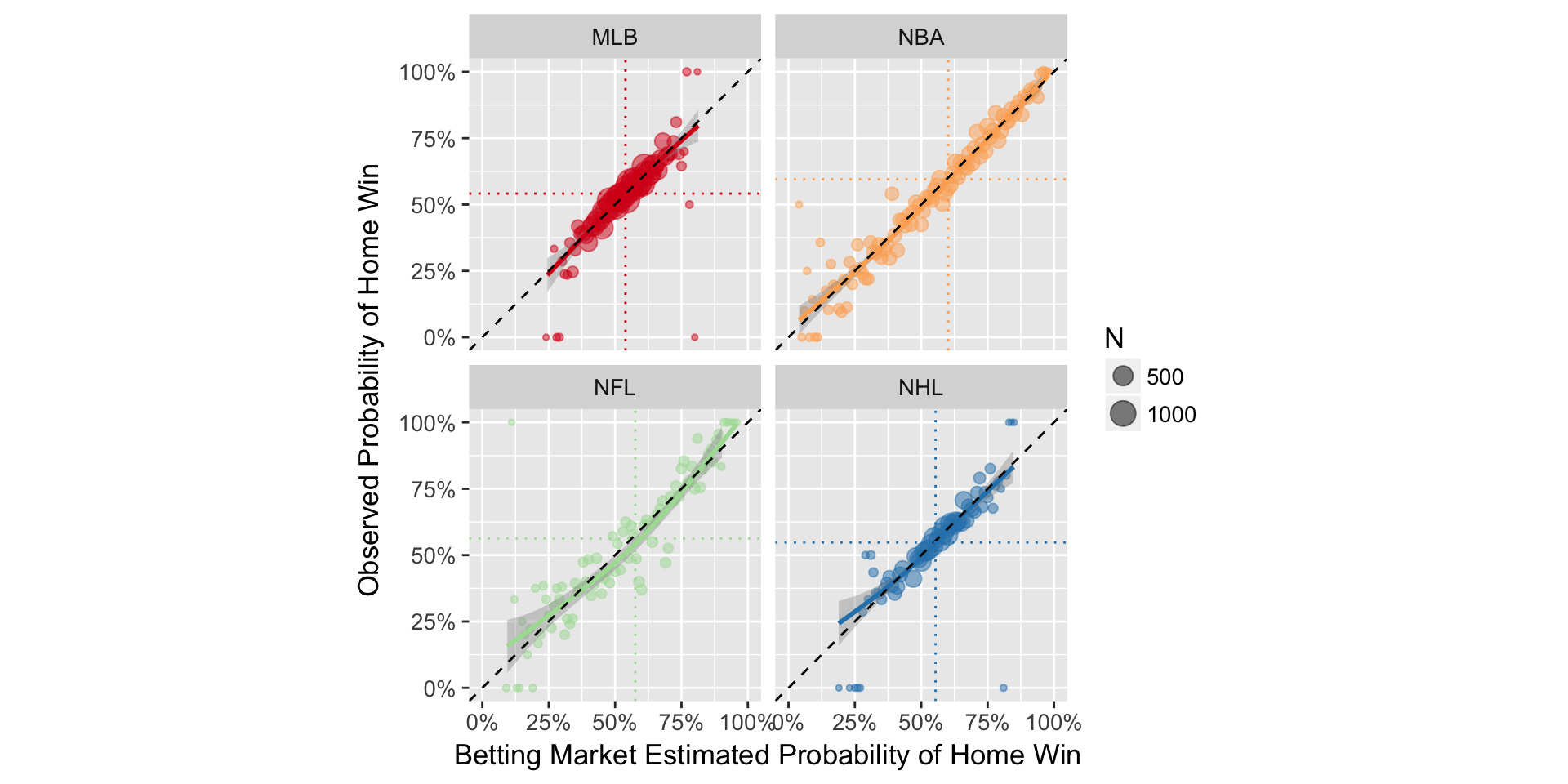## The model: definitions

• $$p_{(q,s,k)ij} =$$ probability that team $$i$$ will beat team $$j$$ in season $$s$$ during week $$k$$ of sports league $$q$$, for $$q \in \{MLB, NBA, NFL, NHL\}$$.
• $$\alpha_{q_{0}}$$ be the league-wide home advantage (HA) in $$q$$
• $$\alpha_{(q) i^{\star}}$$ be the extra effect (+ or -) for team $$i$$ when playing at home
• $$\theta_{(q,s,k) i}$$ and $$\theta_{(q, s, k) j}$$ be season-week team strength parameters

## The model & assumptions

$E[\text{logit}(p_{(q,s,k) ij})] = \theta_{(q,s,k) i} - \theta_{(q, s, k) j} + \alpha_{q_0} + \alpha_{(q) i^{\star}}$

Assumptions:

1. $$\sum_{i=1}^{t_{q}} \theta_{(q,s,k)i} = 0$$
2. $$E[\theta_{(i,q,s+1,1)}] = \gamma_{q, season} \theta_{(i, q,s,k)}$$
3. $$E[\theta_{(i,q,s,k+1)}] = \gamma_{q, week} \theta_{(i, q,s,k)}$$
4. $$\gamma_{q,week}$$ and $$\gamma_{q,season}$$ week/season level autogressive parameters

## Fitting a cross-sport model

• Data
• 2006–2016 reg. season in MLB, NBA, NFL, NHL (Sports Insights)
• Priors
• Uniform (variance parameters) and Normal (team strength parameters)
• Software
• rjags package in R statistical software
• Draws
• 20k iterations, 2k burn in, thin of 5

## Results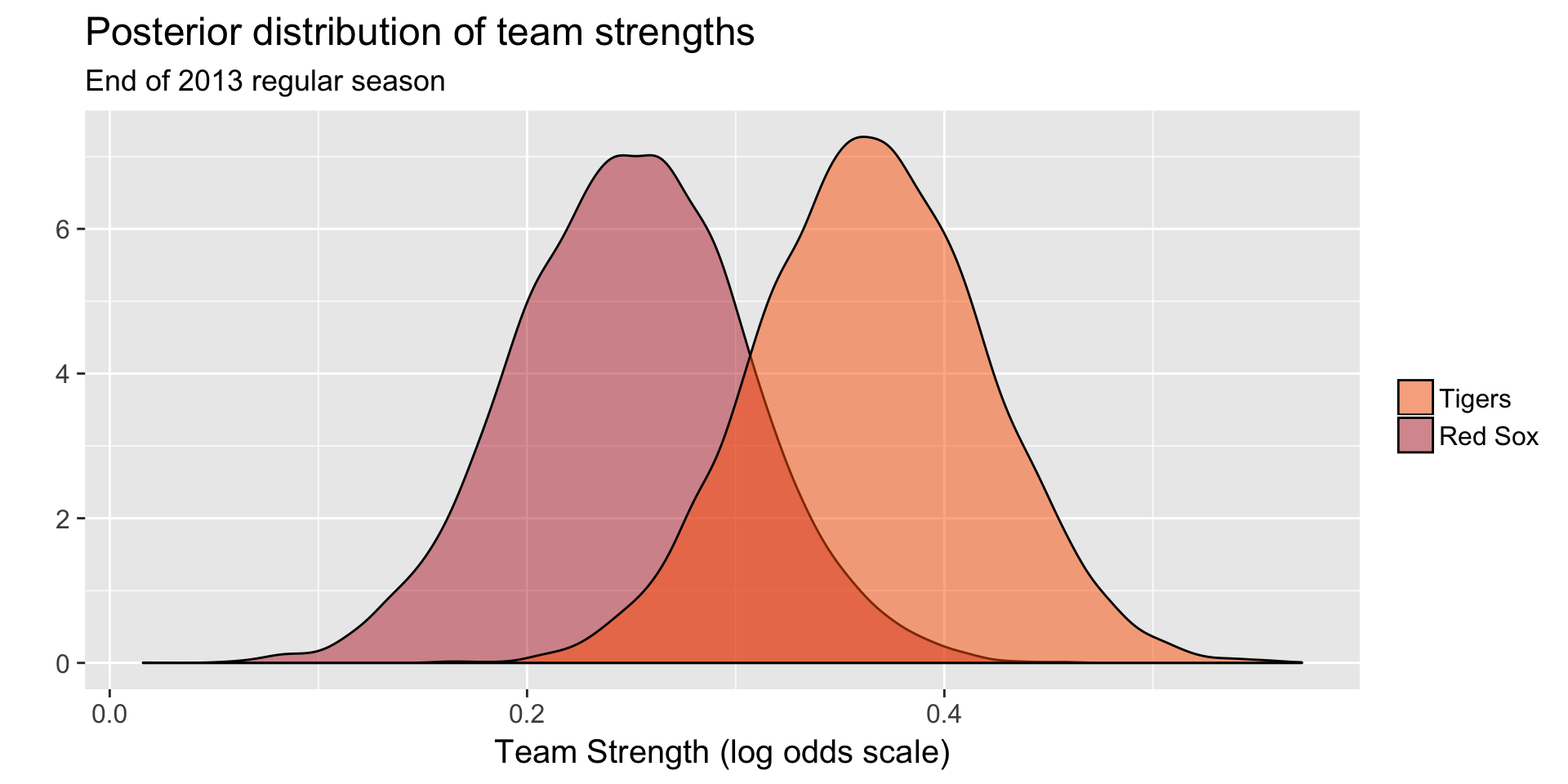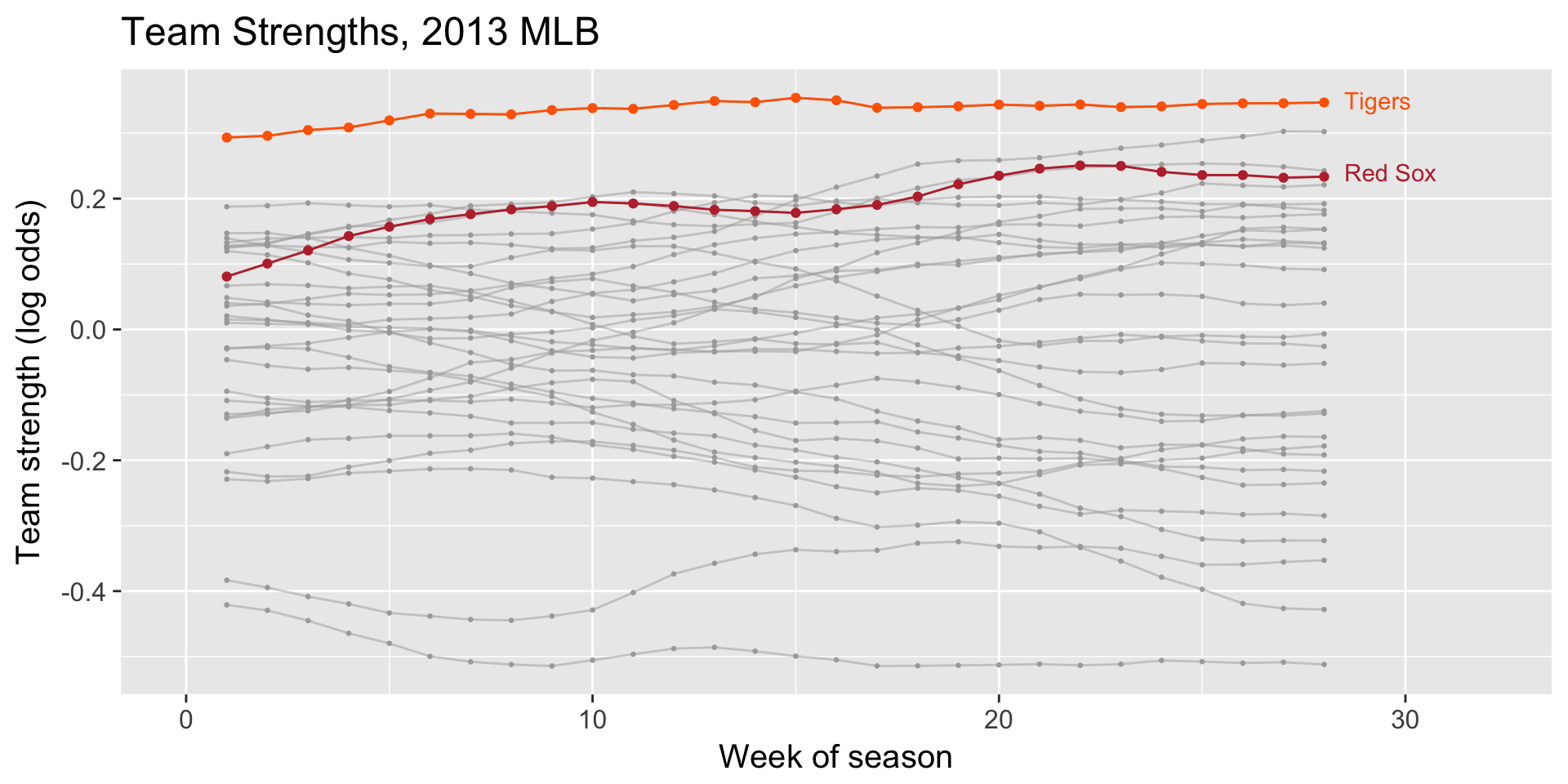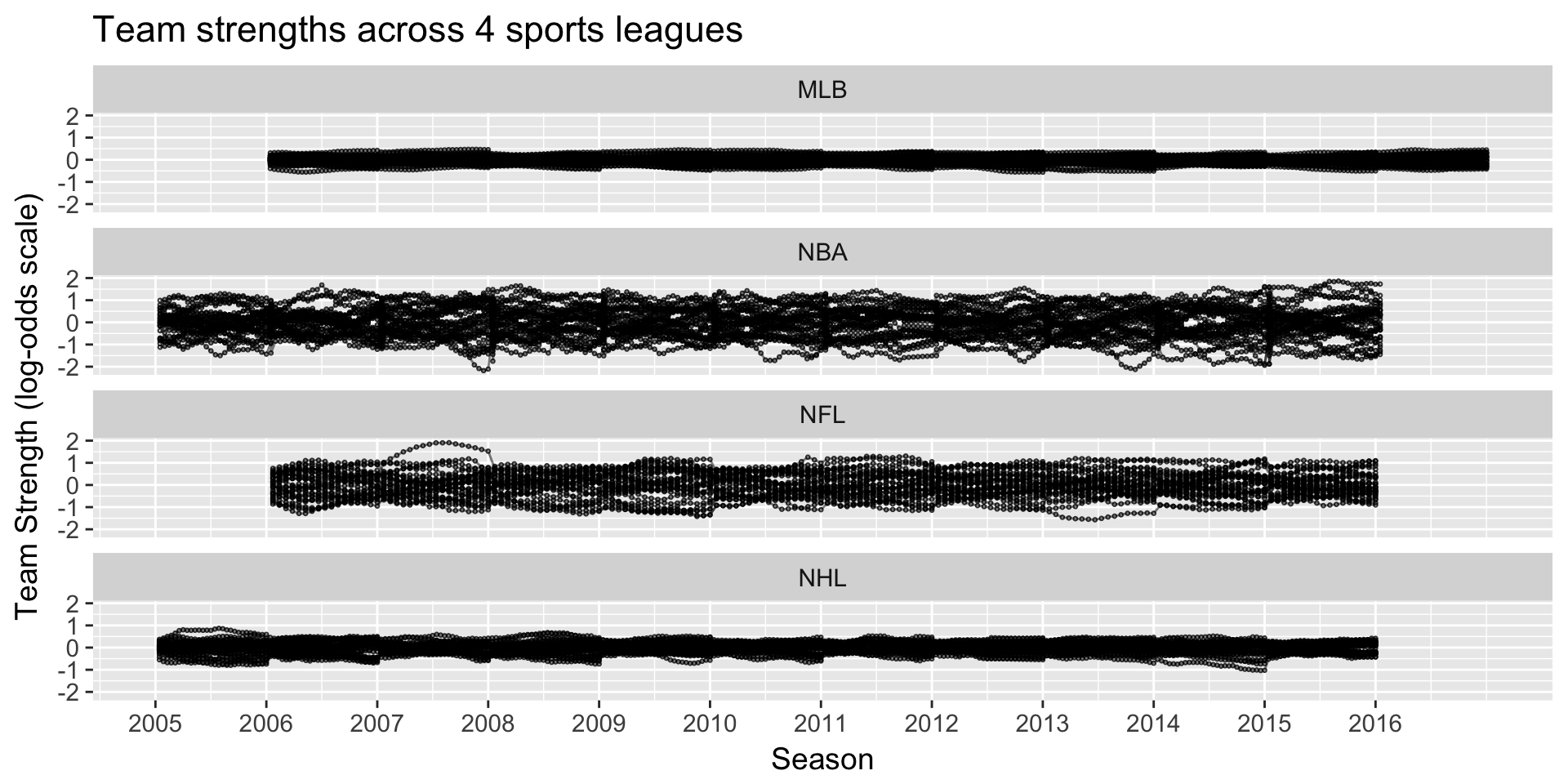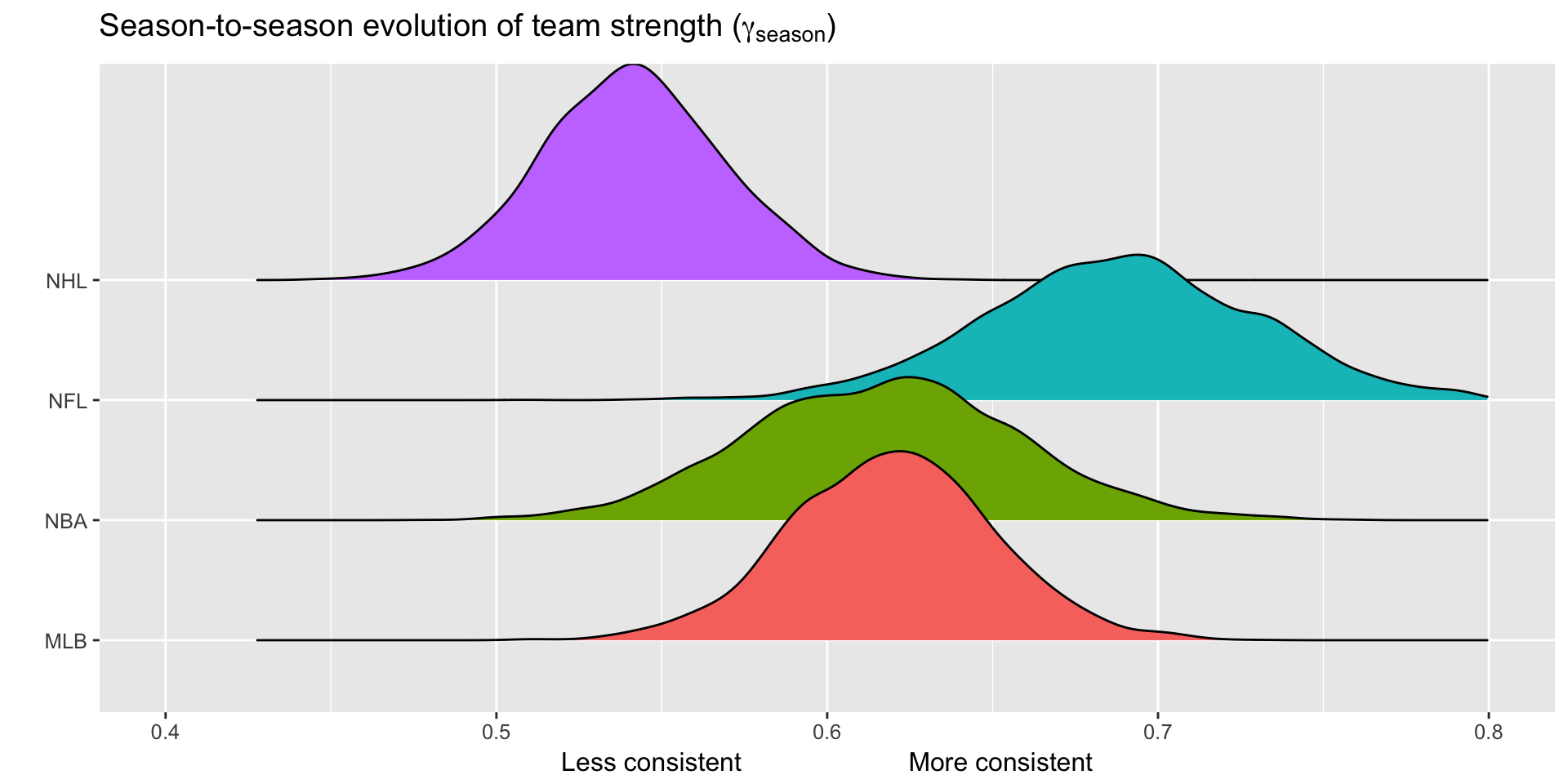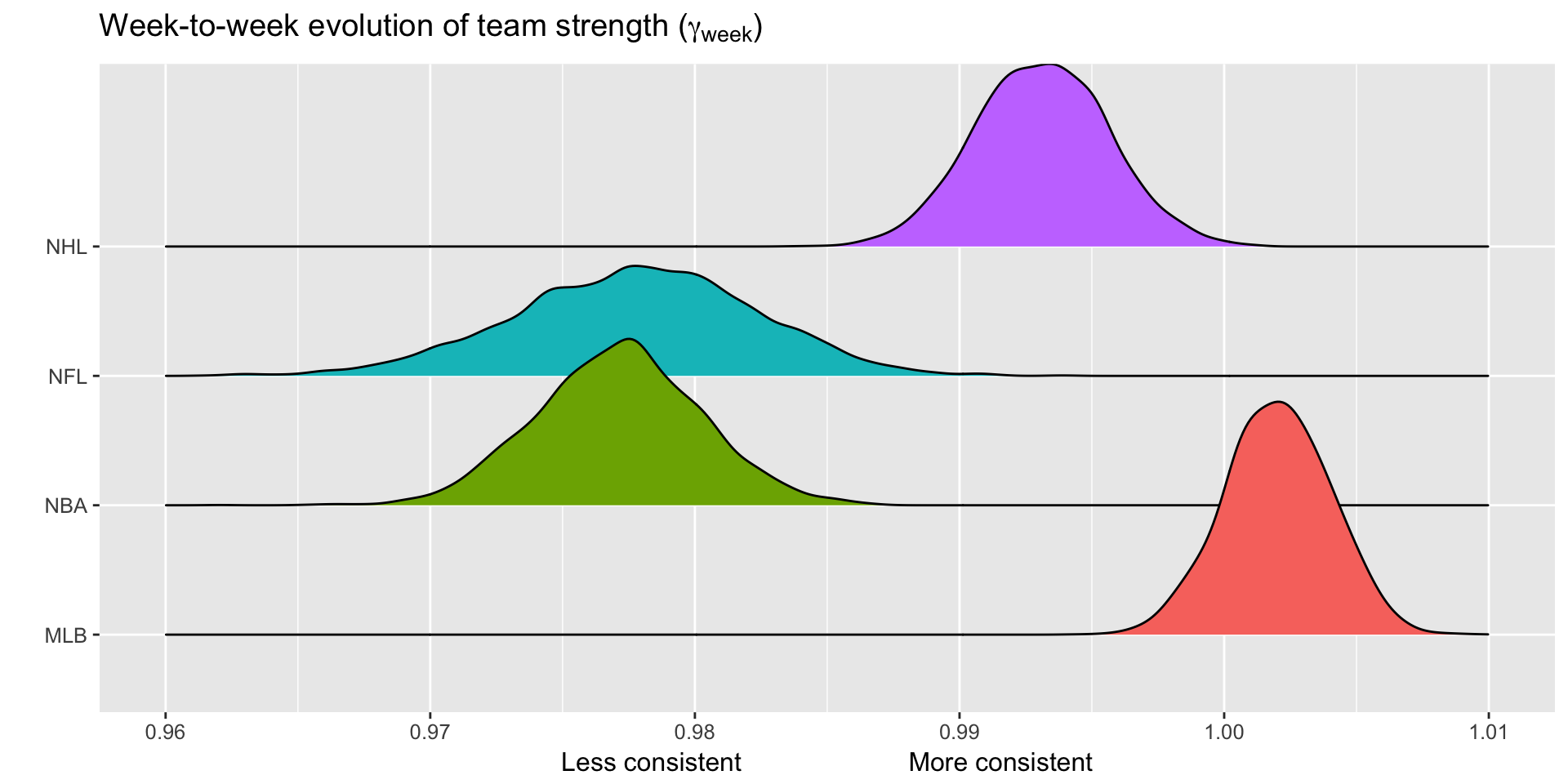## Unpredictability at a fixed point in time

How often does the best team win?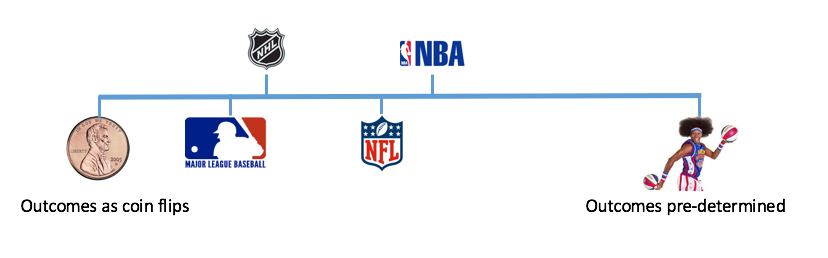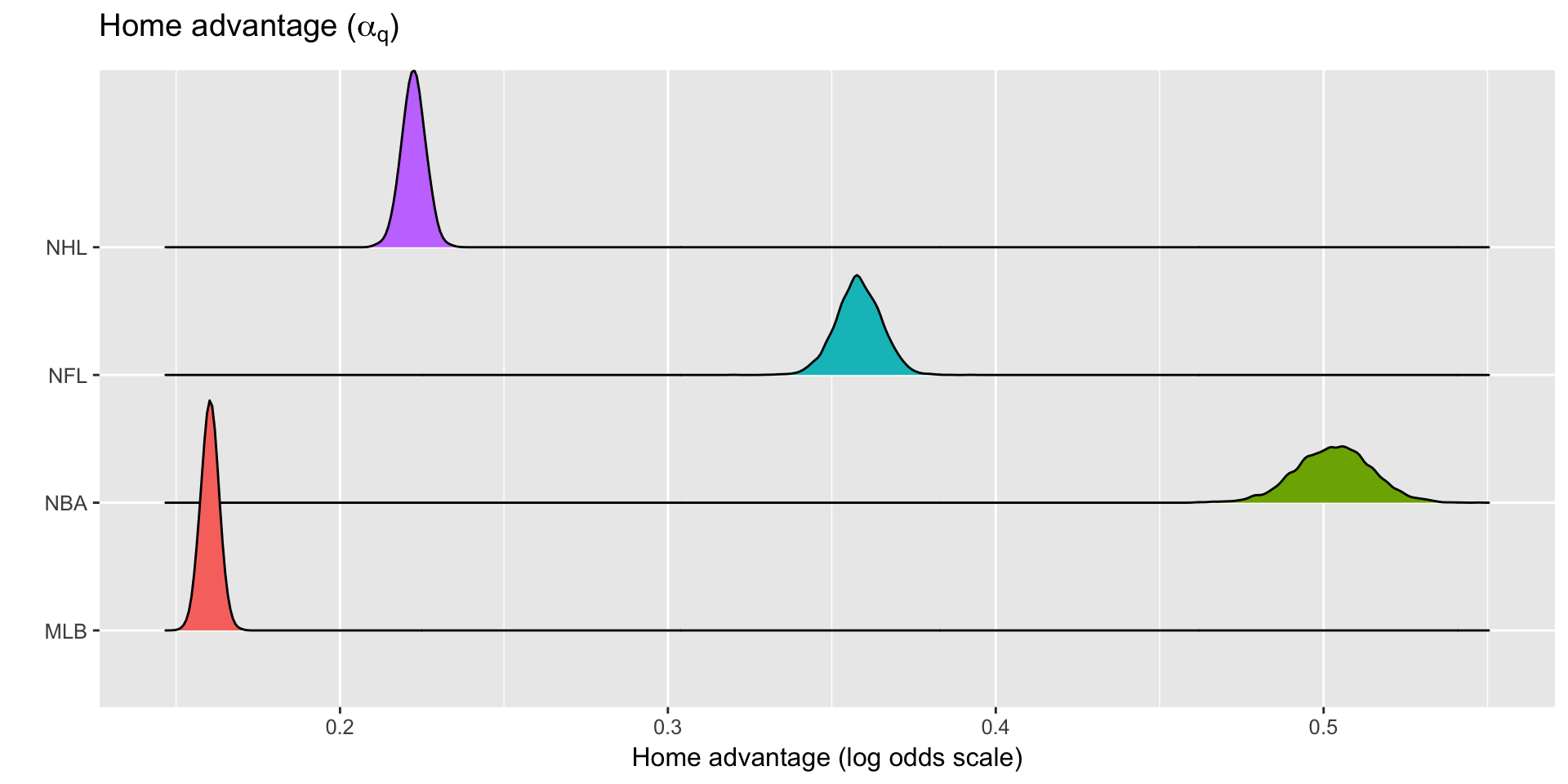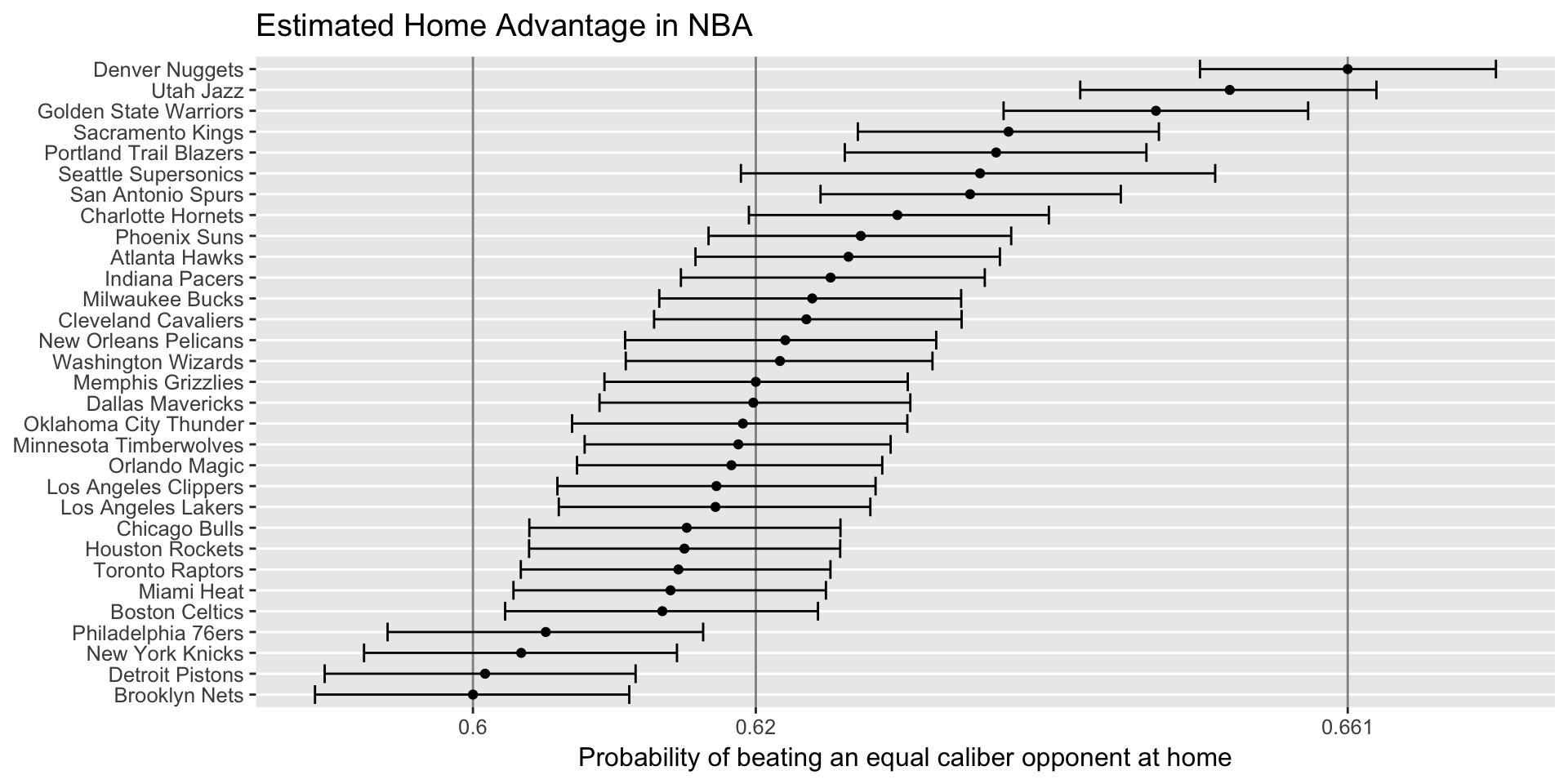## GMs need to predict the future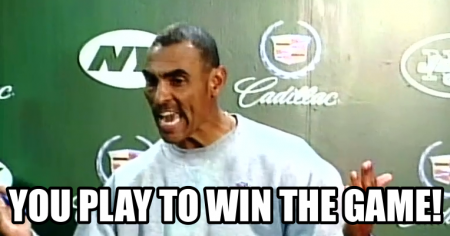## GMs need to strategize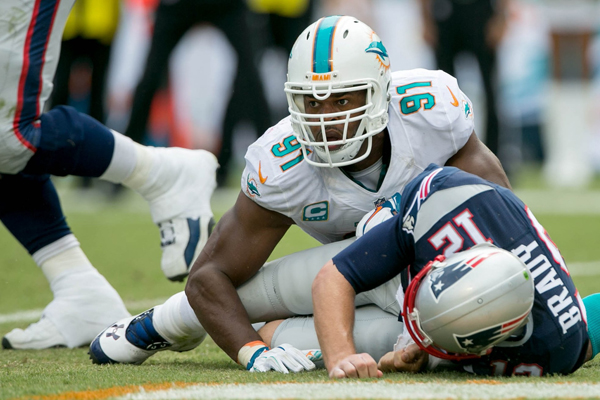## GMs need long term plans

“They have to rethink their whole philosophy”

Mike Milbury to the 2017 Washington Capitals after a playoff series loss to Pittsburgh

## Acknowledgements: Greg